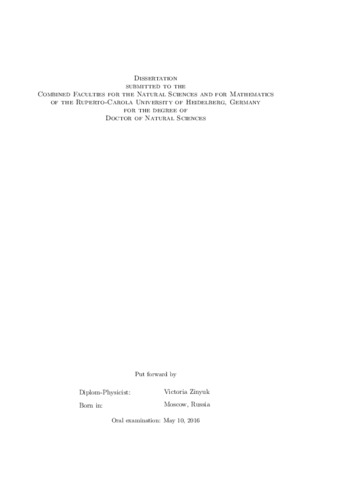## Systematic study of strangeness production and dynamic behaviour with the FOPI experiment at SIS-18

Zinyuk, VictoriaPreview
PDF, English
This work is dedicated to the investigation of in-medium behaviour of strange particles. In-medium production and propagation of $K^+$ and $K^-$ mesons is studied with the FOPI apparatus in $^{58}$Ni\,+\,$^{58}$Ni collisions at the incident beam energy of $E_{beam}$= 1.91 AGeV, i.e. near the strangeness production threshold energies. Weak in-plane anti\,-\,flow' with respect to protons and a slight squeeze-out' is found in the azimuthal emission pattern of $K^{+}$ mesons. $K^{-}$ mesons exhibit an isotropic emission pattern within large statistic uncertainties. The comparison to the predictions of two independent transport calculations, HSD and IQMD, suggests a weakly repulsive $KN$ in-medium potential of $U_{KN} = 20 \pm 5$\,MeV and an attractive $\bar{K}N$ in\,-\,medium potential of $U_{K^{-}N} = -40 \pm 10$\,MeV. Production of $K^{+/-/0}$, $\phi$ mesons and the $\Lambda$ baryon at normal nuclear matter density is studied in $\pi^-+A$ reactions with C, Cu and Pb targets at incident beam momentum of $p_{\pi^-}$= 1.7 GeV/$c$. Differences in the final state phase space distributions measured in heavy and light targets point to repulsion for $K^+$ and $K^0_S$ mesons in nuclear medium. The latter is solely attributed to the strong interaction. The corresponding comparison for $K^-$ mesons suggests a strong absorption in nuclear medium. Observed $\phi$ meson yields support the hypothesis of $Z^{\alpha}$\,-\,scaling of the production cross section.